Generate 3 Unique Random numbers in Microsoft Excel

If you want to generate a list of unique random numbers then you can use RANDBETWEEN functions & VBA RND. In this article, we will generate 3 unique numbers from the provided list.

You can find original question here

Question: I want a macro to generate list of 3 random numbers from a list of numbers saved in column A. There is a condition that VBA code needs to be taken care of. The condition is there are 3 numbers that exist in column B, hence macro needs to create a list of numbers that have not occurred once in column B. In this way, we can have a list of unique numbers in column B & C.

Following is the snapshot of example that we have: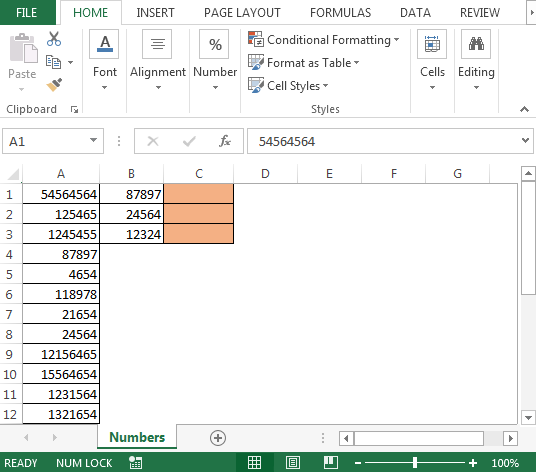To get the list of unique random numbers; we need to follow the below steps to launch VB editor

• Click on Developer tab
• From Code group select Visual Basic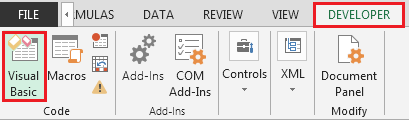• Copy the below code in the standard module

```Sub RandomNumbers()
Dim ws As Worksheet
Dim ar As Variant
Dim RandomNum As Long
Dim i As Integer
Dim myVal As Long
Randomize
Set ws = ThisWorkbook.Sheets("Numbers")
With ws
ar = .Range("A" & Rows.Count).End(xlUp).Row
.Range("C1:C3").ClearContents
For i = 1 To 3
Do
RandomNum = Int((1 - ar + 1) * Rnd + ar)
myVal = .Range("A" & RandomNum).Value
Loop Until Range("B1:C24").Find(what:=myVal, lookat:=xlWhole) Is Nothing
.Range("C" & i).Value = myVal
Next i
End With
End Sub
```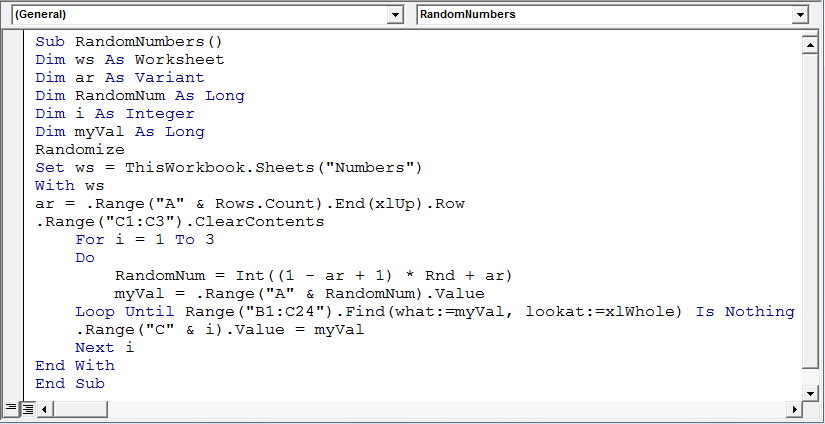• To run the macro, we can press F5 key from keyboard if you are active in Visual Basic Editor’s screen
• Another way to run the macro is using “ALT + F8” key; select the macro & click on Run button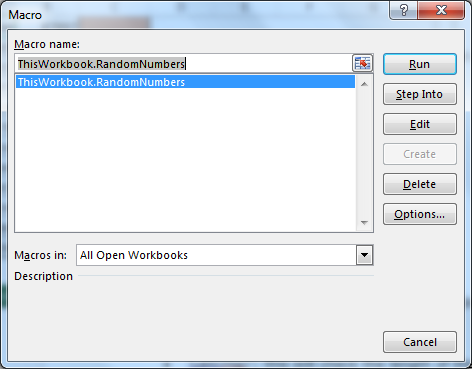• As we run the VBA code, the unique list of numbers will be automatically generated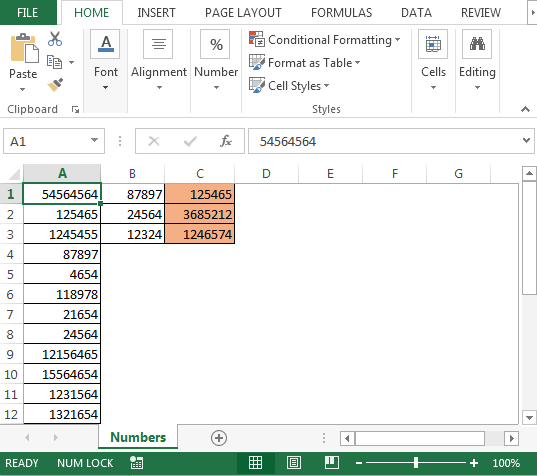Code Explanation:

In the above code, we will use RND & INT function along with For loop & Do loop to get the result.

• We declare Dim ws as Worksheet; the purpose of declaring ws as Worksheet is we only want the code to run on a specific worksheet i.e. Numbers in our example
• Dim RandomNum As Long: this will save the unique numbers
• Set ws = ThisWorkbook.Sheets(“Numbers”): this line will ensure that the code runs on Numbers sheet only
• The next line of code will use With statement
• ar = .Range(“A” & Rows.Count).End(xlUp).Row: this line will save the last cell in column A (24 in our example)
• .Range(“C1:C3″).ClearContents: this line will erase any existing content in range C1:C3
• Next, we will run For loop starting from 1 to 3 times with Do Loop to generate list of random numbers & checking no duplicates in existing range i.e. column B

Conclusion: We can generate list of distinct random numbers from a list in Microsoft excel with small tweaking in the above VBA code.If you liked our blogs, share it with your friends on Facebook. And also you can follow us on Twitter and Facebook.

We would love to hear from you, do let us know how we can improve, complement or innovate our work and make it better for you. Write us at info@exceltip.com

Terms and Conditions of use

The applications/code on this site are distributed as is and without warranties or liability. In no event shall the owner of the copyrights, or the authors of the applications/code be liable for any loss of profit, any problems or any damage resulting from the use or evaluation of the applications/code.